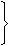# Aptitude - Chain Rule

## Why Aptitude Chain Rule?

In this section you can learn and practice Aptitude Questions based on "Chain Rule" and improve your skills in order to face the interview, competitive examination and various entrance test (CAT, GATE, GRE, MAT, Bank Exam, Railway Exam etc.) with full confidence.

## Where can I get Aptitude Chain Rule questions and answers with explanation?

IndiaBIX provides you lots of fully solved Aptitude (Chain Rule) questions and answers with Explanation. Solved examples with detailed answer description, explanation are given and it would be easy to understand. All students, freshers can download Aptitude Chain Rule quiz questions with answers as PDF files and eBooks.

## Where can I get Aptitude Chain Rule Interview Questions and Answers (objective type, multiple choice)?

Here you can find objective type Aptitude Chain Rule questions and answers for interview and entrance examination. Multiple choice and true or false type questions are also provided.

## How to solve Aptitude Chain Rule problems?

You can easily solve all kind of Aptitude questions based on Chain Rule by practicing the objective type exercises given below, also get shortcut methods to solve Aptitude Chain Rule problems.

### Exercise :: Chain Rule - General Questions

1.

3 pumps, working 8 hours a day, can empty a tank in 2 days. How many hours a day must 4 pumps work to empty the tank in 1 day?

 A. 9 B. 10 C. 11 D. 12

Explanation:

Let the required number of working hours per day be x.

More pumps, Less working hours per day (Indirect Proportion)

Less days, More working hours per day (Indirect Proportion)

 Pumps 4 : 3:: 8 : x Days 1 : 24 x 1 x x = 3 x 2 x 8x = (3 x 2 x 8) (4)x = 12.

2.

If the cost of x metres of wire is d rupees, then what is the cost of y metres of wire at the same rate?

A.
 Rs.xyd
B. Rs. (xd)
C. Rs. (yd)
D.
 Rs.ydx

Explanation:

Cost of x metres = Rs. d.

 Cost of 1 metre = Rs.dx

 Cost of y metres = Rs.d . y= Rs.yd. x x

3.

Running at the same constant rate, 6 identical machines can produce a total of 270 bottles per minute. At this rate, how many bottles could 10 such machines produce in 4 minutes?

 A. 648 B. 1800 C. 2700 D. 10800

Explanation:

Let the required number of bottles be x.

More machines, More bottles (Direct Proportion)

More minutes, More bottles (Direct Proportion)

 Machines 6 : 10:: 270 : x Time (in minutes) 1 : 46 x 1 x x = 10 x 4 x 270x = (10 x 4 x 270) (6)x = 1800.

4.

A fort had provision of food for 150 men for 45 days. After 10 days, 25 men left the fort. The number of days for which the remaining food will last, is:

A.
 29 1 5
B.
 37 1 4
C. 42
D. 54

Explanation:

After 10 days : 150 men had food for 35 days.

Suppose 125 men had food for x days.

Now, Less men, More days (Indirect Proportion)125 : 150 :: 35 : x125 x x = 150 x 35x = 150 x 35 125x = 42.

5.

39 persons can repair a road in 12 days, working 5 hours a day. In how many days will 30 persons, working 6 hours a day, complete the work?

 A. 10 B. 13 C. 14 D. 15

Explanation:

Let the required number of days be x.

Less persons, More days (Indirect Proportion)

More working hours per day, Less days (Indirect Proportion)

 Persons 30 : 39:: 12 : x Working hours/day 6 : 530 x 6 x x = 39 x 5 x 12x = (39 x 5 x 12) (30 x 6)x = 13.